NCERT Exemplar - MCQs

Chapter 6 Class 12 Application of Derivatives
Serial order wise

## (C) decreasing in ((-π)/2 ", "  π/2)  `          (D) decreasing  in ("0, "  π/2)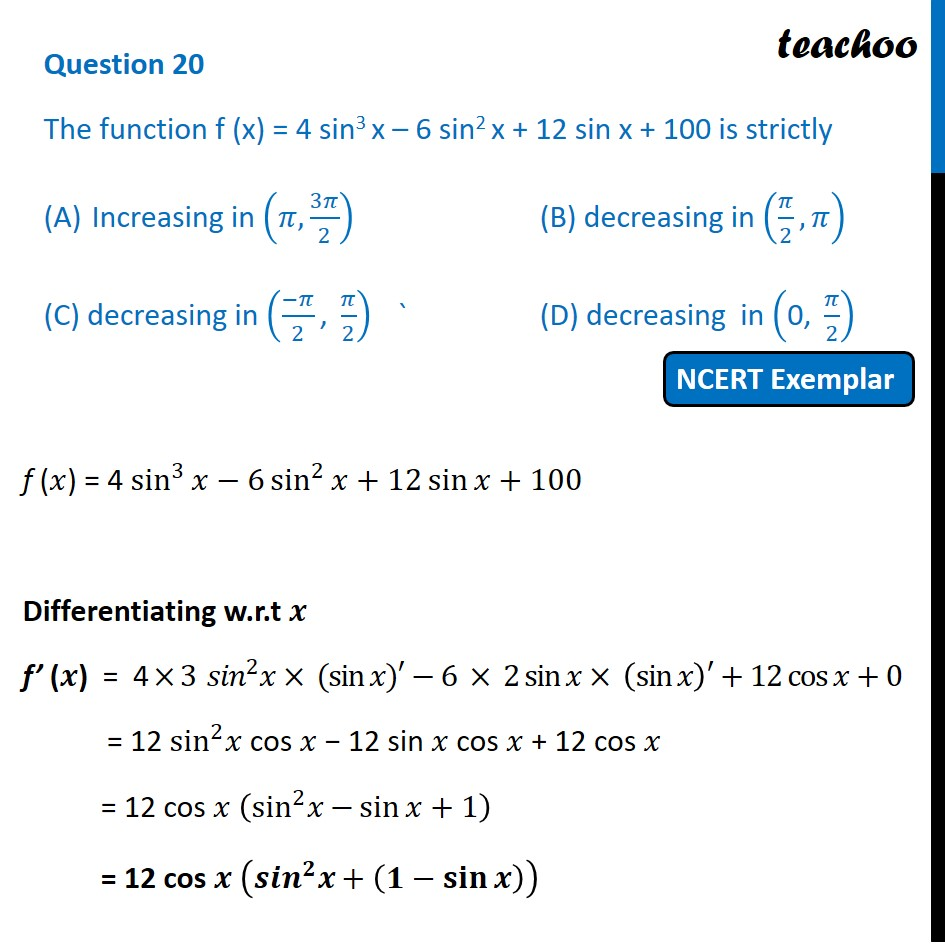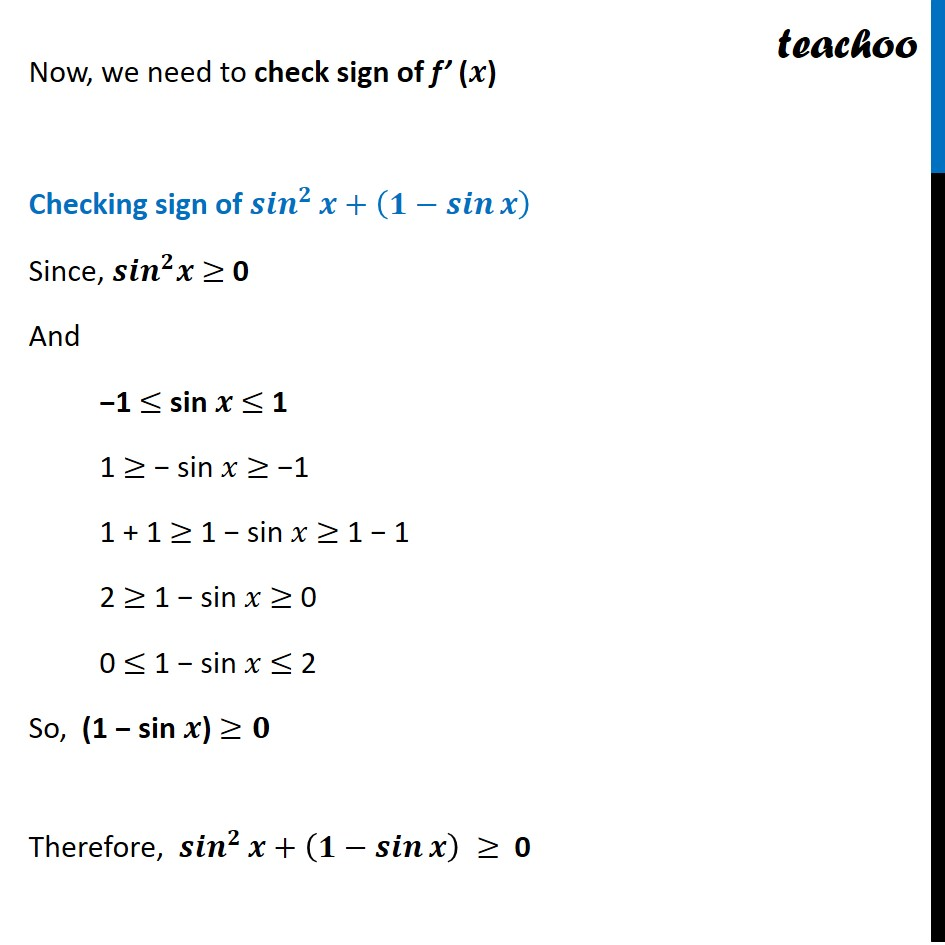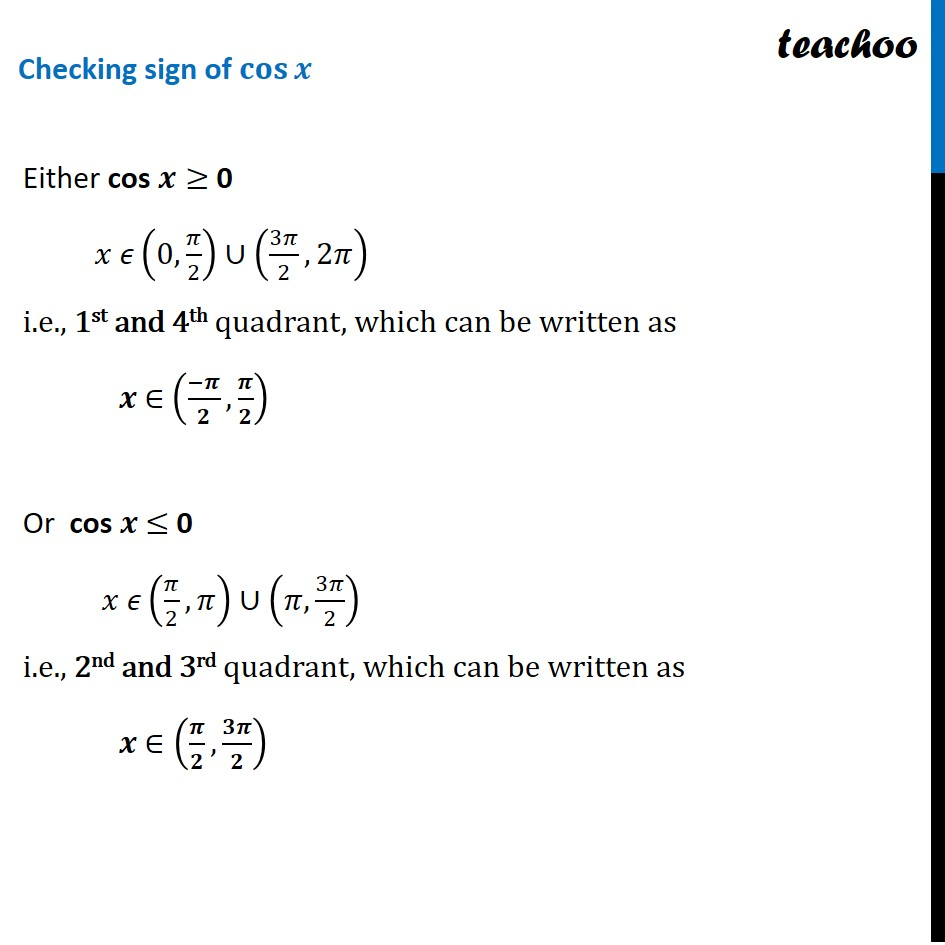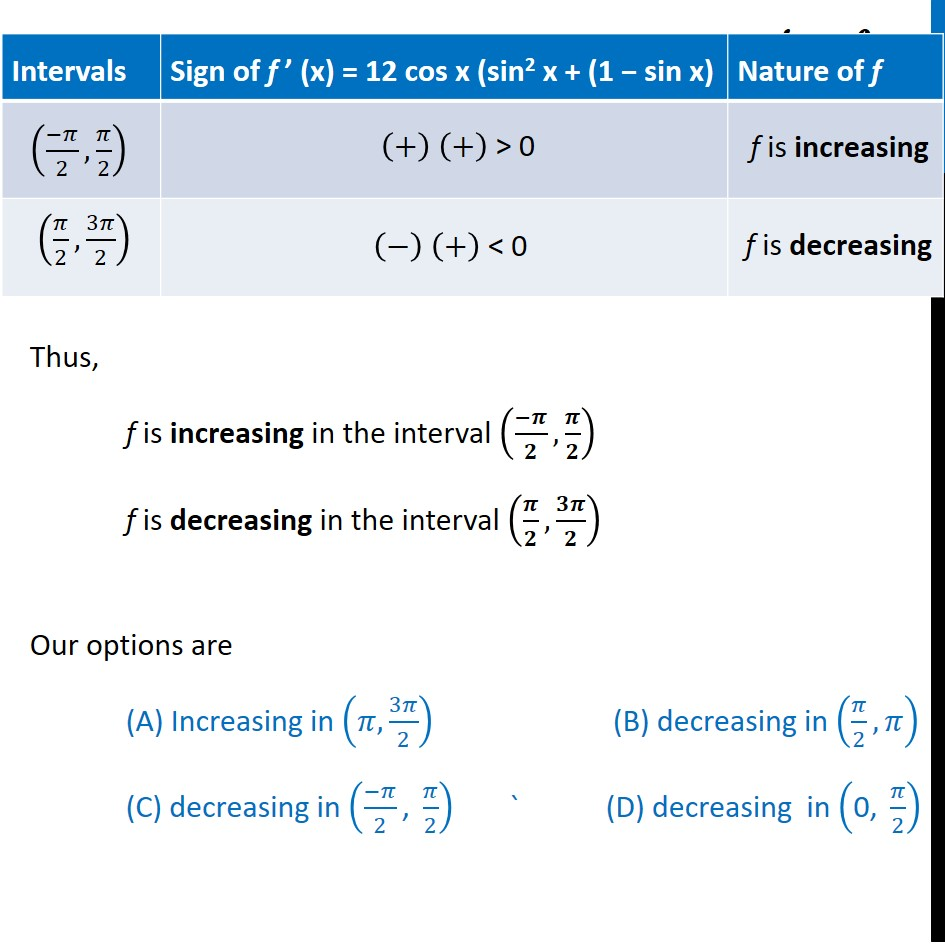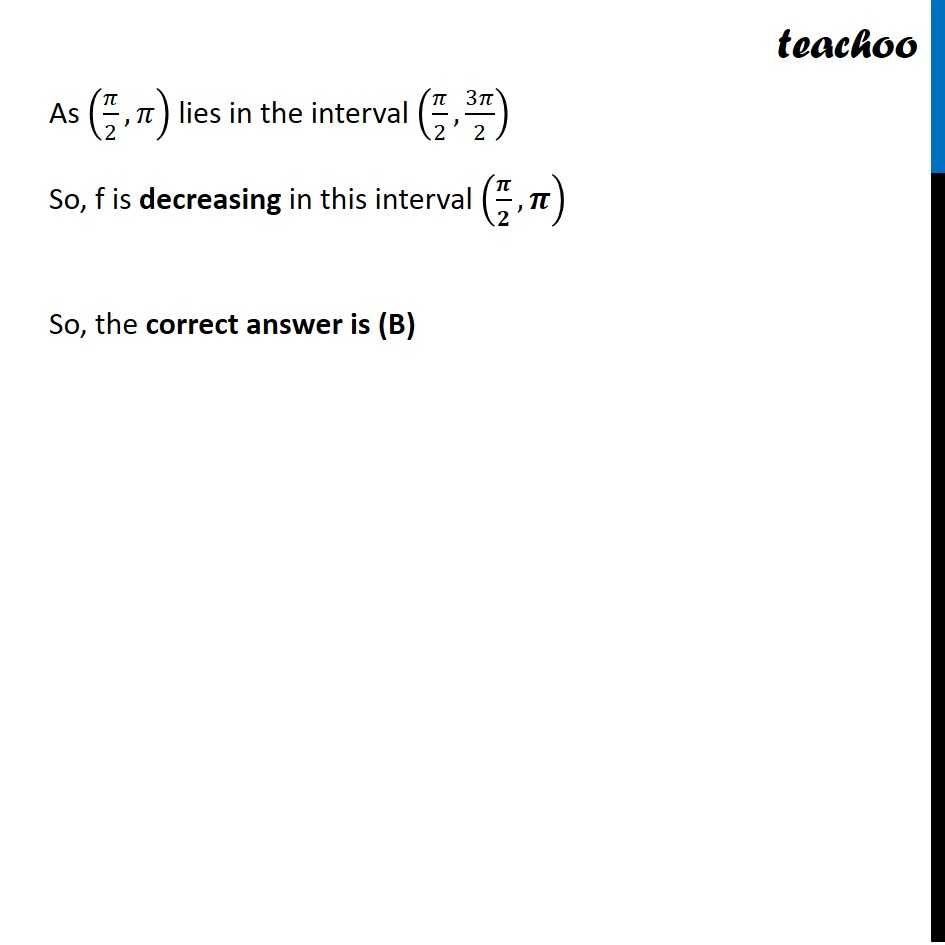Get live Maths 1-on-1 Classs - Class 6 to 12

### Transcript

Question 20 The function f (x) = 4 sin3 x – 6 sin2 x + 12 sin x + 100 is strictly Increasing in (𝜋, 3𝜋/2) (B) decreasing in (𝜋/2,𝜋) (C) decreasing in ((−𝜋)/2 ", " 𝜋/2) ` (D) decreasing in ("0, " 𝜋/2) f (𝑥) = 4 sin^3⁡〖𝑥−6 sin^2⁡〖𝑥+12 sin⁡〖𝑥+100〗 〗 〗 Differentiating w.r.t 𝒙 f’ (𝒙) = 4 ×⁡〖3 〗⁡〖〖𝑠𝑖𝑛〗^2 𝑥〗 × (sin⁡〖𝑥)′〗−6 × 2 sin⁡𝑥× (sin⁡𝑥 )′+12 cos⁡𝑥+0 = 12 sin^2 𝑥 cos 𝑥 − 12 sin 𝑥 cos 𝑥 + 12 cos 𝑥 = 12 cos 𝑥 (〖sin^2 𝑥〗⁡〖−sin⁡〖𝑥+1〗 〗 ) = 12 cos 𝒙 (〖〖𝒔𝒊𝒏〗^𝟐 𝒙〗⁡〖+ (𝟏−𝐬𝐢𝐧⁡𝒙 )〗 ) Now, we need to check sign of f’ (𝒙) Checking sign of 〖𝒔𝒊𝒏〗^𝟐⁡〖𝒙+(〖𝟏−𝒔𝒊𝒏〗⁡𝒙 ) 〗 Since, 〖𝒔𝒊𝒏〗^𝟐 𝒙 ≥ 0 And −1 ≤ sin 𝒙 ≤ 1 1 ≥ − sin 𝑥 ≥ −1 1 + 1 ≥ 1 − sin 𝑥 ≥ 1 − 1 2 ≥ 1 − sin 𝑥 ≥ 0 0 ≤ 1 − sin 𝑥 ≤ 2 So, (1 − sin 𝒙) ≥𝟎 Therefore, 〖𝒔𝒊𝒏〗^𝟐⁡〖𝒙+(〖𝟏−𝒔𝒊𝒏〗⁡𝒙 ) 〗≥ 0 Either cos 𝒙≥ 0 𝑥 𝜖(0, 𝜋/2)∪(3𝜋/2, 2𝜋) i.e., 1st and 4th quadrant, which can be written as 𝒙 ∈ ((−𝝅)/𝟐,𝝅/𝟐) Or cos 𝒙 ≤ 0 𝑥 𝜖(𝜋/2,𝜋)∪(𝜋,3𝜋/2) i.e., 2nd and 3rd quadrant, which can be written as 𝒙 ∈(𝝅/𝟐,𝟑𝝅/𝟐) Thus, f is increasing in the interval ((−𝝅)/𝟐,𝝅/𝟐) f is decreasing in the interval (𝝅/𝟐,𝟑𝝅/𝟐) Our options are (A) Increasing in (𝜋, 3𝜋/2) (B) decreasing in (𝜋/2,𝜋) (C) decreasing in ((−𝜋)/2 ", " 𝜋/2) ` (D) decreasing in ("0, " 𝜋/2) As (𝜋/2,𝜋) lies in the interval (𝜋/2,3𝜋/2) So, f is decreasing in this interval (𝝅/𝟐,𝝅) So, the correct answer is (B)